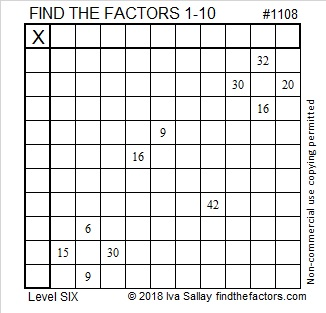# 1108 and Level 6

This level 6 puzzle has eleven clues. Which ones give away the most logical place for you to start it? I hope you have a lot of fun solving this one!Print the puzzles or type the solution in this excel file: 10-factors-1102-1110

Now I’ll share some facts about the number 1108:

• 1108 is a composite number.
• Prime factorization: 1108 = 2 × 2 × 277, which can be written 1108 = 2² × 277
• The exponents in the prime factorization are 1 and 2. Adding one to each and multiplying we get (2 + 1)(1 + 1) = 3 × 2  = 6. Therefore 1108 has exactly 6 factors.
• Factors of 1108: 1, 2, 4, 277, 554, 1108
• Factor pairs: 1108 = 1 × 1108, 2 × 554, or 4 × 277
• Taking the factor pair with the largest square number factor, we get √1108 = (√4)(√277) = 2√277 ≈ 33.286631108 is the hypotenuse of a Pythagorean triple:
460-1008-1108 which is 4 times (115-252-277)

1108 is a palindrome when it is written in three other bases:
It’s 454 in BASE 16 because 4(16²) + 5(16) + 4(1) = 1108,
3E3 in BASE 17 (E is 14 base 10) because 3(17²) +14(17) +3(1) = 1108, and
1E1 in BASE 27 because 27² + 14(27) + 1 = 1108